Several times I stumbled into the fact that the memory access patterns are important for the efficiency. I understand the reasoning behind it, but how much are they really important? Do you get a percent faster execution or ten times faster execution? In this article, we would like to find the answers to these questions.

We will explain a couple of memory access patterns and compare them between each other.

## Matrices

Typical example for studying memory access patterns is a matrix. For all non-mathematicians out there, the matrix is just a rectangular array of numbers.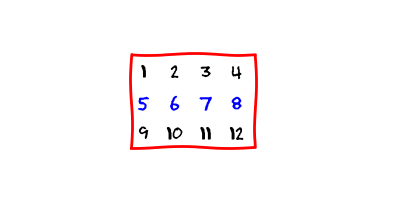We store a matrix in a contiguous chunk of memory. There are two ways to do it. One way is to store the matrix in the row-wise format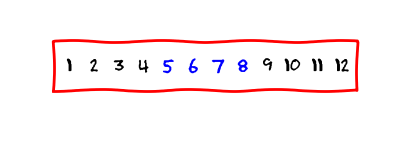and the other way is to store the matrix in the column-wise format.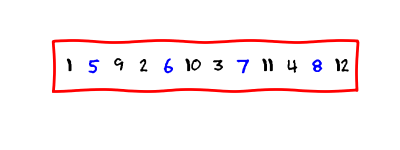We choose to use the row-wise approach.

## Computer’s memory

A computer has a hierarchical memory system. The slowest memory is the main memory. Here, the computer stores data for the program. In our example, the whole matrix would be stored in the main memory.

Then there are other types of memory, which are smaller, faster and closer to the processors. These memories are collectively known as a cache.

A processor can only access the data which is already in the cache. If the data is not there, then the computer copies the data from the main memory into the cache.

The cache has a smaller size as the main memory. Therefore, the computer can not store the whole main memory there. However, the computer usually stores a contiguous chunk of memory. An example of such behavior is presented below.

## The main memory, the cache and matrices

Let us return back to our example. We have the matrix.The matrix is stored in the row-wise format.Let us access the second element in the first row in the matrix (the number `2`). The computer transfers the data into the cache (we assume that the computer can fit three elements into it):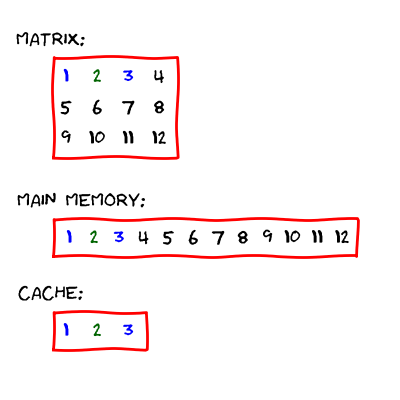Then, the processor is able to access the element.

But, what if we now access the third element in the first row (the number `3`)? The computer first looks if the element is already in the cache. It is a lucky day, the element is already there! The processor can directly access the element.

But, what if we would like to access the third element in the second row (the number `6`)? This element in currently not in the cache. Therefore, the computer updates it. The new situation is displayed below.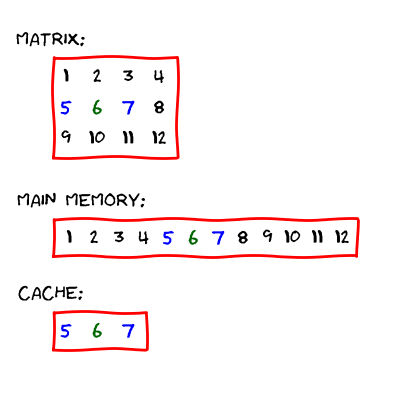Now the processor can access the element.

We see that the same operation (accessing the element in the matrix) has potentially different overheads. If the element is not in the cache, the computer must perform additional operations.

Of course, changing of the cache is unavoidable in a program. But an inefficient program changes it more frequently than an efficient program. Let again consider a `n` times `n` matrix `a` with row-wise storage. The task is to sum all the elements in the matrix. The first approach

is more efficient regarding the cache than the second version

In the second example, the computer changes the cache every time when we access the element (if `n` is higher than the size of the cache). But this does not happen in the first example. There, the cache does not change so often.

An important fact is that we are not able to directly program the cache memory access. We can only be aware about it and consider it in our programs. But sometimes, we might indirectly influence it as in the examples above.

## Is it really a difference?

Does described memory access patterns really matter so much? Let us make an experiment.

We implement the matrix class with the row-wise storage. Then we compute the sum of all elements in the matrix. The program sums the elements two times: in the row-wise and in the column-wise for loop (like in the examples above). We measure the time needed for the row-wise and the column-wise summation. The entire source code is available here.

The results are presented below.

In the first column are the numbers of elements in the matrix. Second column displays the time (in microseconds) for the row-wise summation. The third column shows the time for the column-wise summation. The last column presents the ratio between the column-wise and the row-wise summation. If the ratio is equal to ten, then the row-wise summation is ten times faster than the column-wise summation.

The results are quite surprising to me. I did not expect such a difference between the two approaches. In some cases the row-wise version outperforms the column-wise version by a factor of 10. This is the difference between a quadratic and a linear algorithm. This is very big!

What can we learn from this example? Well, the memory access patterns are important! If you are programming a performance critical software, then you should be aware of them and you should use them in your advantage!

## Summary

We learned the differences between the different memory access patterns. We examined their performance. The conclusion is that they are important and we should be aware of them.